Question

4. For the beam and loading shown, draw the shear force and bending moment diagrams and...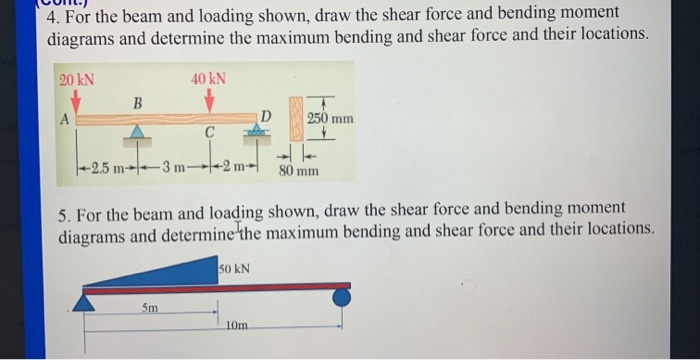4. For the beam and loading shown, draw the shear force and bending moment diagrams and determine the maximum bending and shear force and their locations. 20 KN 40 KN B D 250 mm |--2.5 m- 3m-4-2 m 80 mm 5. For the beam and loading shown, draw the shear force and bending moment diagrams and determine the maximum bending and shear force and their locations. 50 KN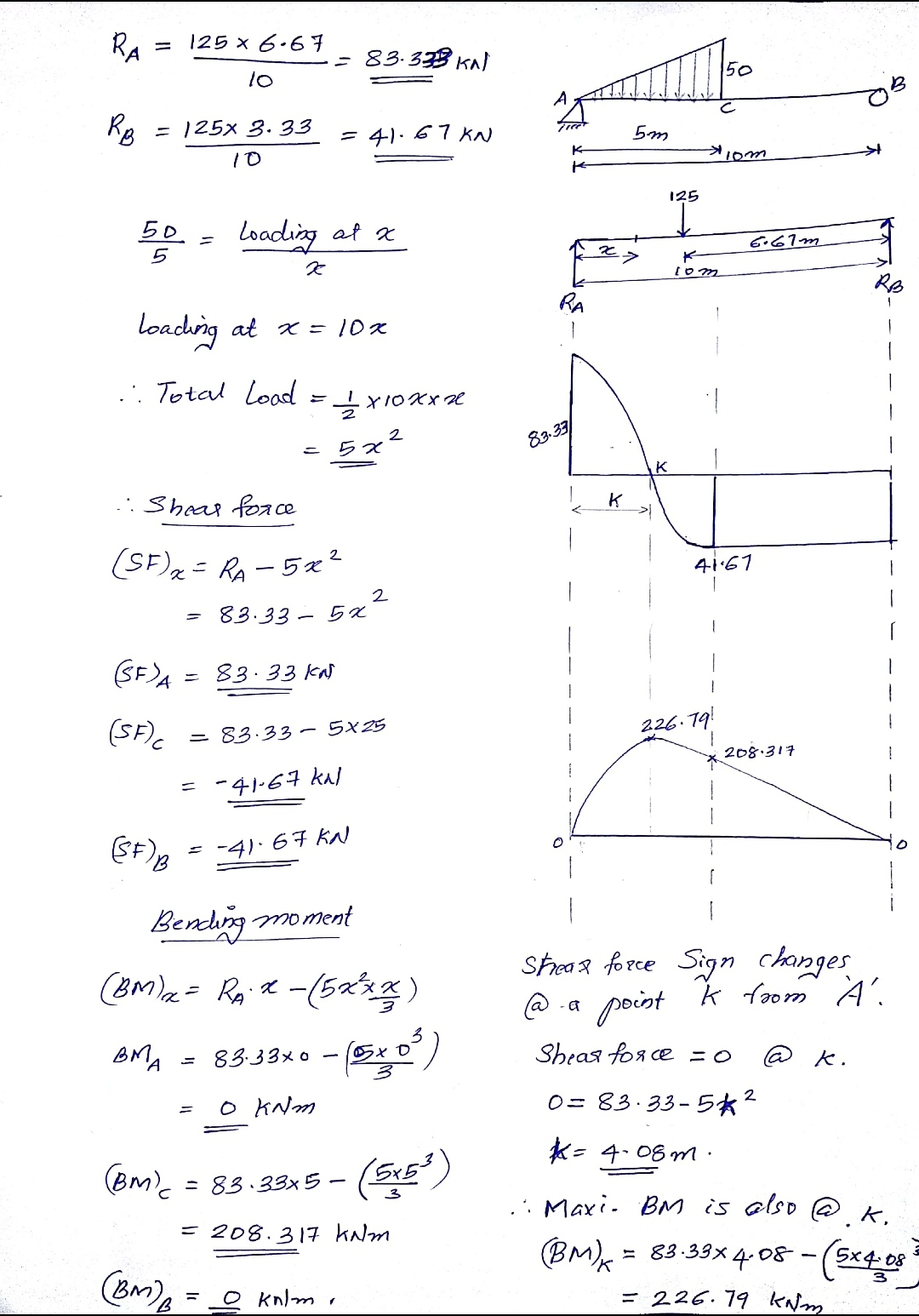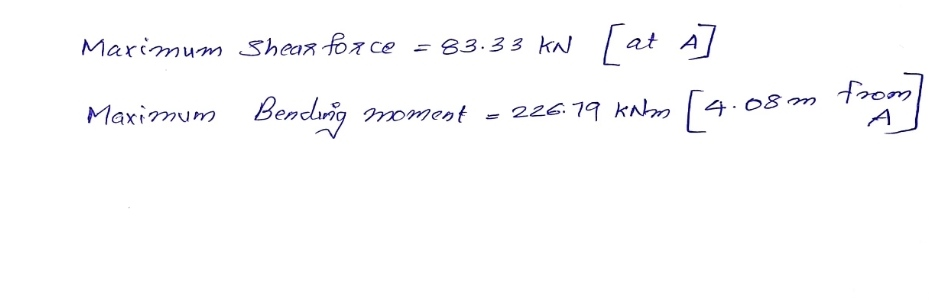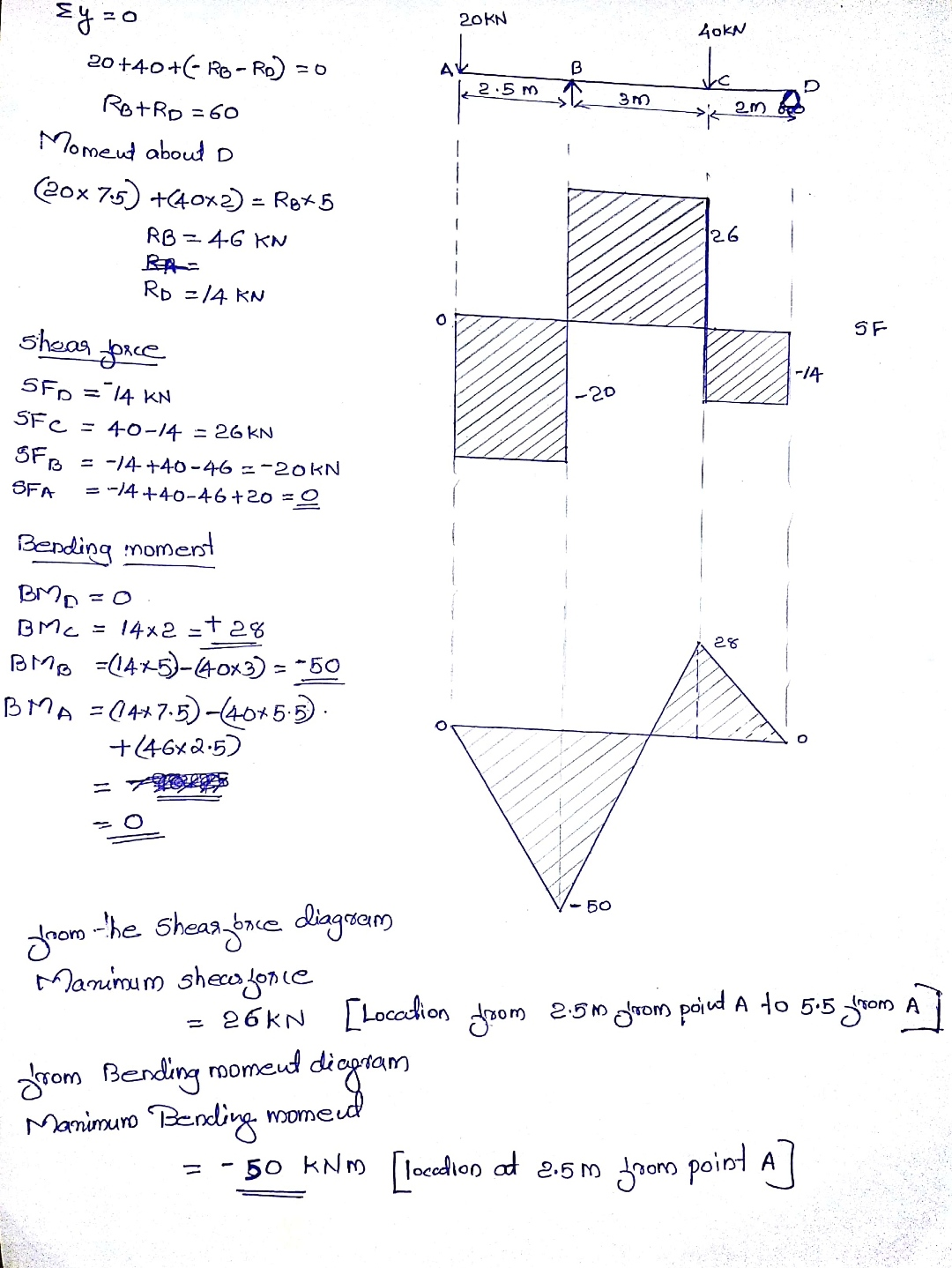Earn Coins

Coins can be redeemed for fabulous gifts.

Similar Homework Help Questions
• Draw the shear and bending-moment diagrams for the beam and loading shown and determine the maximum...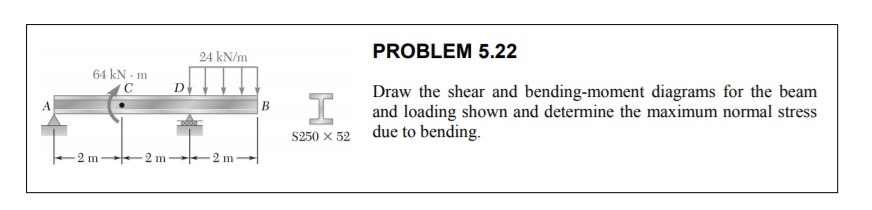Draw the shear and bending-moment diagrams for the beam and loading shown and determine the maximum normal stress due to bending. 24 kN/m PROBLEM 5.22 64 kNm Draw the shear and bending-moment diagrams for the beam and loading shown and determine the maximum normal stress due to bending. \$250 X 52 2 m2 m2 m--

• Draw the Shear Force and Bending Moment Diagrams for the beam and loading shown. P =...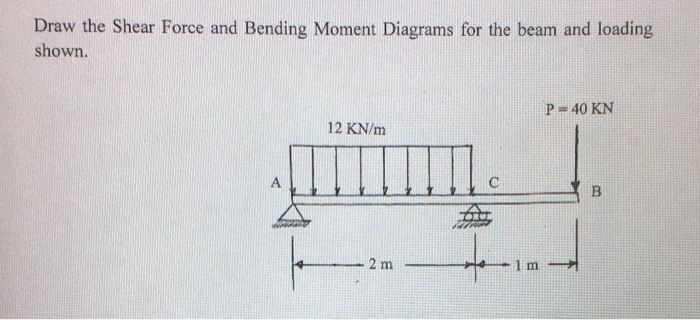Draw the Shear Force and Bending Moment Diagrams for the beam and loading shown. P = 40 KN 12 KN/m В 2 m 1 m

• For the beam and loading shown, draw the shear and bending moment diagrams, and determine the...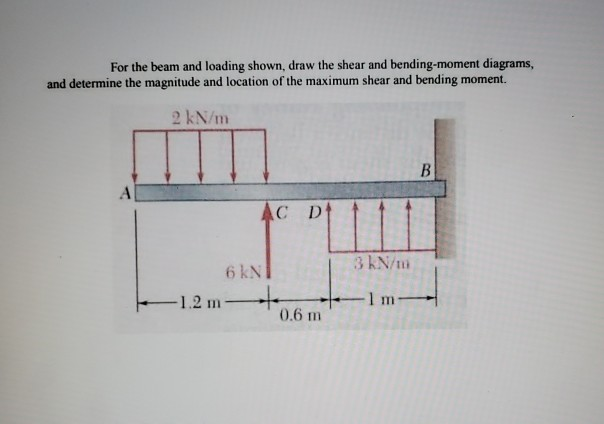For the beam and loading shown, draw the shear and bending moment diagrams, and determine the magnitude and location of the maximum shear and bending moment. 2 kN/m AC D 6 NT 3kN/m lm-- 1.2 m 0.6 m

• d 5.23 Draw the shear and bending-moment diagrams for the beam and loading shown and determine...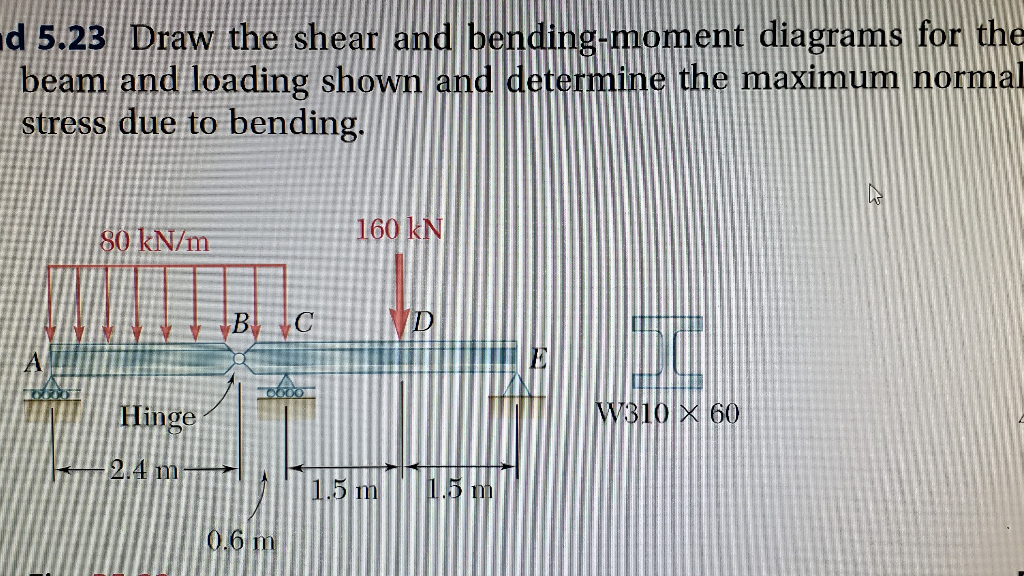d 5.23 Draw the shear and bending-moment diagrams for the beam and loading shown and determine the maximum normal stress due to bending. 160 KN 80 kN/m Hinge MB10 X 60 2.4 m m 1.0 D

• For the beam and loading shown draw the shear and bending moment diagrams and then find...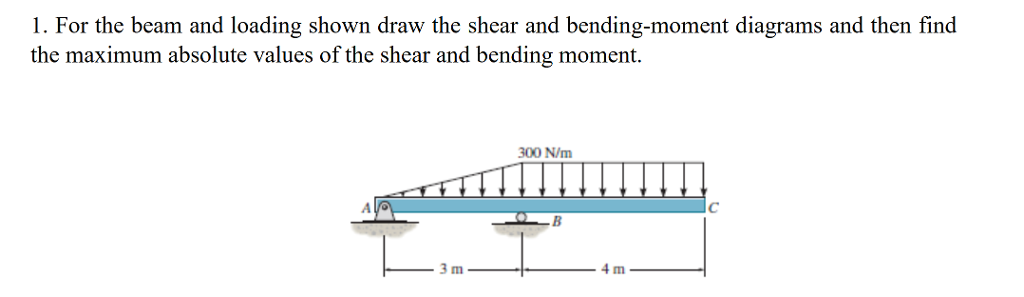For the beam and loading shown draw the shear and bending moment diagrams and then find the maximum absolute values of the shear and bending moment. 1. For the beam and loading shown draw the shear and bending-moment diagrams and then find the maximum absolute values of the shear and bending moment. 300 N/m 4 m

• Draw the shear force and bending moment diagrams of the beam with the span, loading condition...Draw the shear force and bending moment diagrams of the beam with the span, loading condition and cross section. Draw the normal stress and shear stress distributions at the cross section of the beam B structural bearing. www q=77 kN/m b=40 mm h=250 mm t=30 mm 9 В| 9 B TA h 2m 5m 2m 40 b 40

• Problem 1A,B: Draw the shear and bending moment diagrams for the beam and loading shown, and...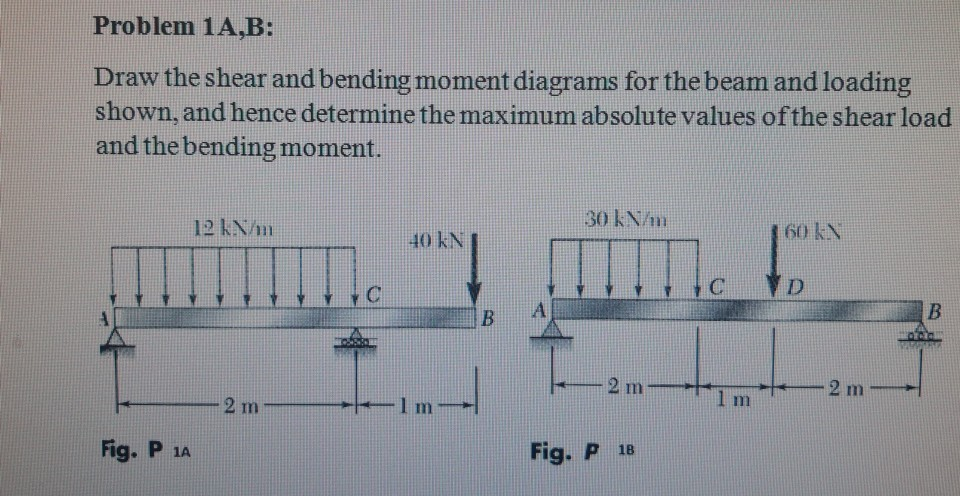Problem 1A,B: Draw the shear and bending moment diagrams for the beam and loading shown, and hence determine the maximum absolute values of the shear load and the bending moment. 30 kN/m 12 km 160 kN D 2 m 2 m 1 m 2 mm Fig. P 12 Fig. P 18

• 7.69 and 7.70For the beam and loading shown, (a) draw the shear and bending-moment diagrams, (b)...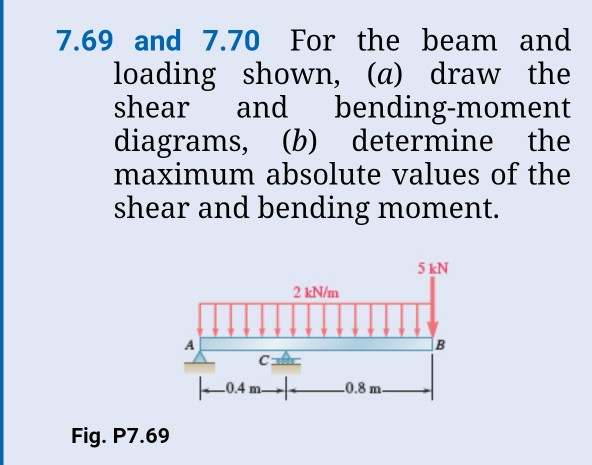7.69 and 7.70For the beam and loading shown, (a) draw the shear and bending-moment diagrams, (b) determine the maximum absolute values of the shear and bending moment. 5 kN 2 kN/m 0.8 m 0.4 Fig. P7.69

• PROBLEM 6 For the beam and loading shown, (a) draw the shear and bending-moment diagrams, (b)...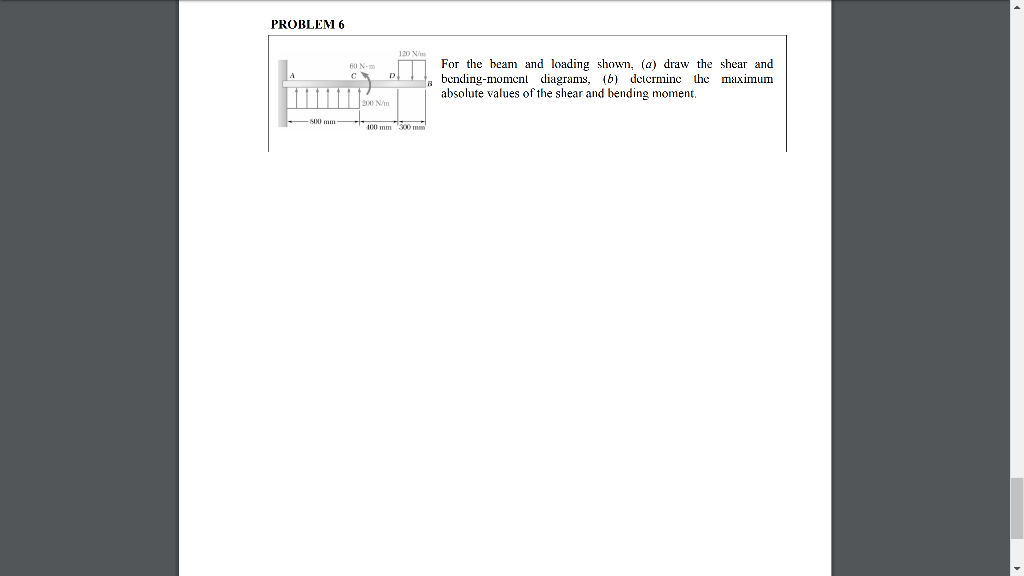PROBLEM 6 For the beam and loading shown, (a) draw the shear and bending-moment diagrams, (b) determine the maximum absolute values of the shear and bending moment. PROBLEM 6 120 W BON For the beam and loading shown, (a) draw the shear and bending-moment diagrams, (6) detcrminc the maximum absolute values of the shear and bending moment, 90 mm 100 mm

• Draw the shear and bending-moment diagrams for the beam and loading shown, and determine the maximum...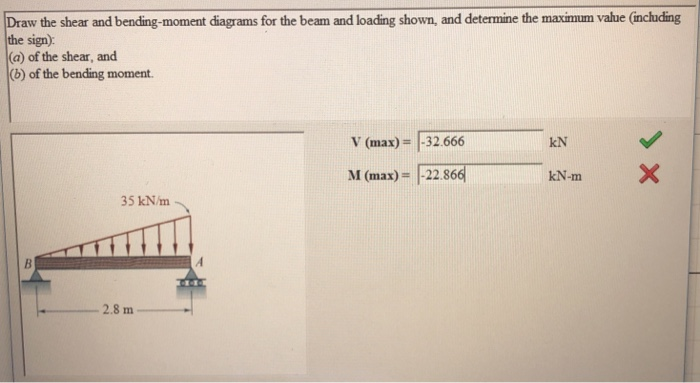Draw the shear and bending-moment diagrams for the beam and loading shown, and determine the maximum value (including the sign) (a) of the shear, and (b) of the bending moment. V (max)- 32 666 M (max)-|-22.86d kN kN-m 35 kN/m 2.8 m